Schumaker Spline

2021-09-09

This package implements the schumaker spline for one dimensional interpolation. This is the first publicly available R package to give a shape-constrained spline without any optimisation being necessary. It also has significant speed advantages compared to the other shape constrained splines. It is intended for use in dynamic programming problems and in other cases where a simple shape constrained spline is useful.

This vignette first illustrates that the base splines can deliver nonconcave splines from concave data. It follows by describing the options that the schumaker spline provides. It then describes a consumption smoothing problem before illustrating why the schumaker spline performs significantly better than existing splines in these applications.

Finally the speed of this spline in comparison with other splines is described.

The schumaker spline and base splines

We can show that base splines are not shape preserving. Plotting the base monotonic spline (you can experiment with the other ones for a similar result):

x = seq(1,10)
y = log(x)

xarray = seq(1,10,0.01)

BaseSpline = splinefun(x,y, method = "monoH.FC")
Base0 = BaseSpline(xarray)
Base1 = DerivBaseSpline(xarray)
Base2 = Deriv2BaseSpline(xarray)

plot(xarray, Base0, type = "l", col = 4, ylim = c(-1,3), main = "Base Spline and first two derivatives", ylab = "Spline and derivatives", xlab = "x")
lines(xarray, Base1, col = 2)
lines(xarray, Base2, col = 3)
abline(h = 0, col = 1)
text(x=rep(8,8,8), y=c(2, 0.5,-0.2), pos=4, labels=c('Spline', 'First Derivative', 'Second Derivative'))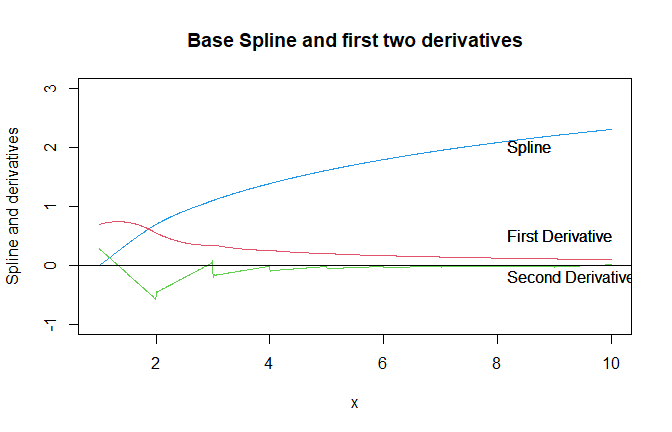Here you can see that the first derivative is always positive - the spline is monotonic. However the second derivative moves above and below 0. The spline is not globally concave.

Now we can show the schumaker spline:

library(schumaker)
SchumSpline = schumaker::Schumaker(x,y)
Schum0 = SchumSpline$Spline(xarray) Schum1 = SchumSpline$DerivativeSpline(xarray)
Schum2 = SchumSpline$SecondDerivativeSpline(xarray) plot(xarray, Schum0, type = "l", col = 4, ylim = c(-1,3), main = "Schumaker Spline and first two derivatives", ylab = "Spline and derivatives", xlab = "x") lines(xarray, Schum1, col = 2) lines(xarray, Schum2, col = 3) abline(h = 0, col = 1) text(x=rep(8,8,8), y=c(2, 0.5,-0.2), pos=4, labels=c('Spline', 'First Derivative', 'Second Derivative'))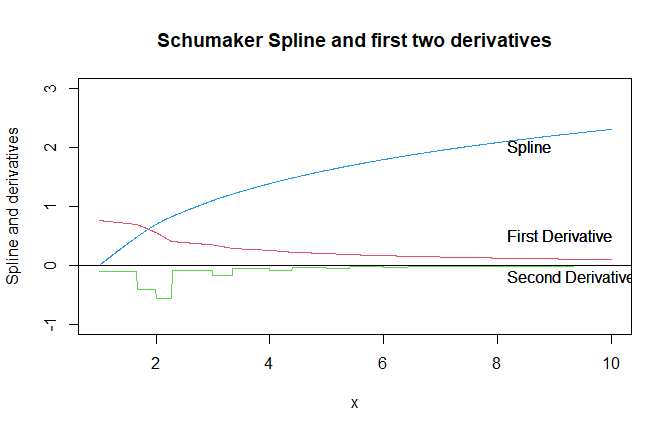Here the second derivative is always negative - the spline is globally concave as well as monotonic. Optional Settings There are three optional setting in creating a spline. Firstly the gradients at each of the (x,y) points can be input to give more accuracy. If not supplied these are estimated from the points. Secondly if Vectorised = TRUE arrays can be input to the spline. If arrays will never be input to the spline then you can set Vectorised = FALSE for a small speed improvement (about 30%). There are three options for out of sample prediction. • Curve - This is where the quadratic curve that is present in the first and last interval are used to predict points before the first interval and after the last interval respectively. • Linear - This is where a line is extended out before the first interval and after the last interval. The slope of the line is given by the derivative at the start of the first interval and end of the last interval. • Constant - This is where the first and last y values are used for prediction before the first point of the interval and after the last part of the interval respectively. The three out of sample options are shown below. Here you can see in black the curve is extended out. In green the ends are extrapolated linearly whilst red has constant extrapolation. Note that there is no difference between the 3 within sample. x = seq(1,10) y = log(x) xarray = seq(-5,15,0.01) SchumSplineCurve = Schumaker(x,y, Extrapolation = "Curve" )$Spline

SchumSplineConstant = Schumaker(x,y, Extrapolation = "Constant")$Spline SchumSplineLinear = Schumaker(x,y, Extrapolation = "Linear" )$Spline

SchumSplineCurveVals    = SchumSplineCurve(xarray)
SchumSplineConstantVals = SchumSplineConstant(xarray)
SchumSplineLinearVals   = SchumSplineLinear(xarray)

plot(xarray, SchumSplineCurveVals, type = "l", col = 1, ylim = c(-5,5),
main = "Ways of predicting outside of sample", ylab = "Spline value", xlab = "x")
lines(xarray, SchumSplineConstantVals, col = 2)
lines(xarray, SchumSplineLinearVals, col = 3)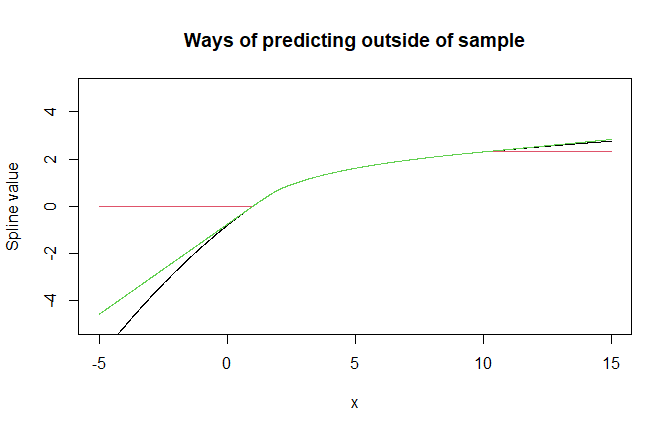Finally there is the possibility to set the edge gradients manually whilst imputing all of the other gradients. This is done through the edgeGradients setting. By default this setting is c(NA,NA) which means that the default gradients specified in Judd (1998) are used. If this setting is set to c(0,NA) this replaces the left side gradient with a zero gradient. Both edge gradients or only the right gradient can be set analogously.

This edgeGradients can be used to solve a nonmonotonicity problem caused by edge segments that only increase or decrease by a small amount. For instance in the below case, gradient imputation causes nonmonotonicity (while convexity is retained). 1

x = c(-3,-1,-0.5,0)
y = c(0, 0.007, 2, 5)

sp_all = Schumaker(x,y)
sp = sp_all$Spline sp1 = sp_all$DerivativeSpline
sp2 = sp_all$SecondDerivativeSpline xarray = seq(min(x), max(x), length.out = 500) yarray0 = sp(xarray) plot(x,y, col = 1) lines(xarray,yarray0, col = 2) yarray1 = sp1(xarray) lines(xarray,yarray1, col = 3) yarray2 = sp2(xarray) lines(xarray,yarray2, col = 4)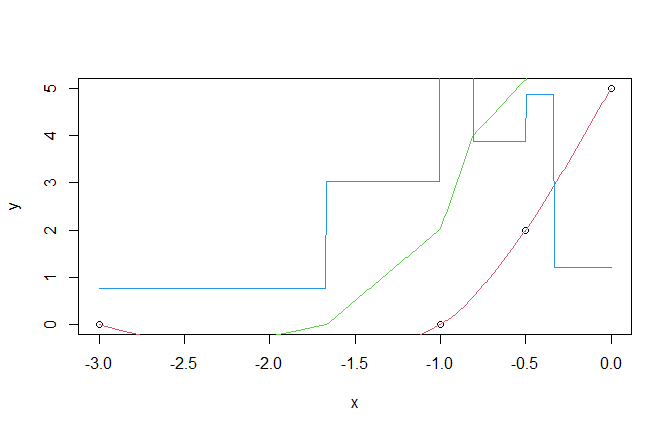This can be fixed by using edgeGradients to set the left side gradient to zero: x = c(-3,-1,-0.5,0) y = c(0, 0.007, 2, 5) sp_all = Schumaker(x,y, edgeGradients = c(0,NA)) sp = sp_all$Spline
sp1 = sp_all$DerivativeSpline sp2 = sp_all$SecondDerivativeSpline

xarray = seq(min(x), max(x), length.out = 500)
yarray0 = sp(xarray)
plot(x,y, col = 1)
lines(xarray,yarray0, col = 2)
yarray1 = sp1(xarray)
lines(xarray,yarray1, col = 3)
yarray2 = sp2(xarray)
lines(xarray,yarray2, col = 4)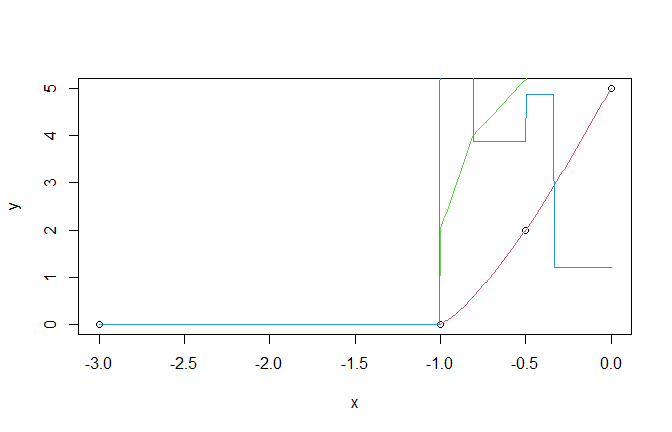Speeding up using multiple functions for interpolations for various groups.

Consider we have a dataframe with x and y variables for multiple different groups. We want to interpolate using this dataframe. We need a different approximation function for each group. The schumaker package implements a way to do this. For every group present in the dataframe a new function is created. A function that calls the correct interpolation function is returned.

As an example consider we have some equity prices for several stocks, several days and several times of the day. We want to be able to interpolate for other times. First generating some example data.

RICs = c("BARC.L", "VOD.L", "IBM.L")
Dates = as.Date(c("11-11-2019", "12-11-2019", "13-11-2019", "14-11-2019", "15-11-2019"), format="%d-%m-%Y")
times = seq(0,28800, length.out = 10) # We are going to interpolate by time of day. This is the x variable. So we state it in terms of seconds. from start of day's trade.
dd = expand.grid(TIME = times, Date = Dates, RIC = RICs)
dd = merge(dd, data.frame(RIC = RICs, PRICE = c(160.00, 162.24, 137.24))) # Making example prices. These are not accurate and I am ignoring currency.
randomness = rlnorm(dim(dd))
dd$PRICE = dd$PRICE * cumprod(randomness)

Now we can create a function that interpolates for each day and for each stock. Then we can intterpolate to get the interpolated price at the desired time of the day. This is done below, first with the base function approxfun and secondly with a schumaker spline.

approx_func = function(x,y){approxfun(x, y)}
dispatched_approxfun = make_approx_functions_from_dataframe(dd, group_vars = c("RIC", "Date"), x_var = "TIME", y_var = "PRICE", approx_func)
dispatched_approxfun("BARC.L", Dates, c(100, 156, 6045))
##  1.392587 1.408422 1.686688
approx_func = function(x,y){Schumaker(x, y)$Spline} approxfun_in_lists = make_approx_functions_from_dataframe(dd, group_vars = c("RIC", "Date"), x_var = "TIME", y_var = "PRICE", approx_func) dispatched_approxfun("IBM.L", Dates, c(100, 156, 6045)) ##  0.039535420 0.039118110 0.004943805 Example: A simple consumption smoothing problem Consider a consumer that has a budget of $$B_t$$ at time $$t$$ and a periodic income of $$1$$. She has a periodic utility function given by: $$u_t = \epsilon_t x_t^{0.2}$$ where $$x_t$$ is spending in period $$t$$ and $$\epsilon_t$$ is the shock in period $$t$$ drawn from some stationary nonnegative shock process with pdf $$f(\epsilon)$$. The problem for the consumer in period $$t$$ is: $$V(B_t | \epsilon_{t}) = \max_{0 < x_t < B} \hspace{0.5cm} \epsilon_t x_t^{0.2} + \beta E_t[ V(B_{t+1})]$$ Where $$\beta$$ is a discounting factor and $$B_{t+1} = 1 + B_t - x_t$$. Algorithm We can first note that due to the shock process it is not possible to get analytical equations to describe the paths of spending and the budget over the long term. We can get a numerical solution however. The key step is to find expressions for the expected value function as a function of $$B_{t+1}$$. With this we can run simulations with random shocks to see the long term distributions of $$x_t$$ and $$B_t$$. The algorithm we will use is: 1. We discretize the budget statespace. 2. We make an initial guess of the future value function $$E[ V(B_{t+1})]$$ at every value of $$B_{t+1}$$ in our discretized statespace. A deterministic approximation of the problem (assume shocks will be $$E[\epsilon_{t}]$$ forever) is often good for this. 3. We use the schumaker spline to join together our estimates at each point in the discretized statespace into an interpolation function. 4. At every point in the statespace we create updated estimates • We use a cubature package to estimate $$\int^\infty_{0} V(B_t | \epsilon_t ) f(\epsilon_t) d\epsilon_t$$ • In this integral we use a one dimensional optimizer along with the above equation to evaluate $$V(B_t | \epsilon_t )$$ 1. Check your convergence criteria. Are the new $$V(B_t | \epsilon_t )$$ values very close to the old values? • If they are we end the algorithm • If they are not we go back to point 3. This strategy relies on the consumption problem being a contraction mapping. This means that if we use this algorithm we will converge to a fixed point function. The FixedPoint package (by the same authors as this package) can be useful here in accelerating the convergence. A more formal presentation of the preeding consumption smoothing problem and the code to solve it can be found in a paper discussing fixed point acceleration in R (Baumann & Klymak 2019). Why a schumaker spline is necessary There are a few reasons we need the spline to be shape preserving and without optimization to make this work: • Shape preservation is necessary so the spline is globally concave (the $$V(B_t | \epsilon_t )$$ values will always be concave as the periodic utility function is always concave). This is necessary for the one dimensional optimisation step. The periodic utility function is concave and the future value function needs to be concave (in $$B_{t+1}$$) to guarantee a unique local optima. Other splines can incorporate tiny convexities in the intervals between interpolation points which can lead to multiple local optima. • There do exist shape preserving splines that incorporate an optimization step (ie scam, cobs). These are not effective for this kind of problem because it is not possible to converge to a fixed point. In each iteration the optimization settles on a slightly different parameter set which means the future value function can “jump around”. In addition the optimization step can take a significant amount of time because evaluations of the future value function spline take longer. Speed • The schumaker spline is of comparable speed to create as other shape preserving splines. • Compared to other splines (including the base spline), the schumaker spline is faster or roughly equally fast in evaluating points. These benchmarks are available below: library(cobs) library(scam) ## Loading required package: mgcv ## Loading required package: nlme ## This is mgcv 1.8-36. For overview type 'help("mgcv-package")'. ## This is scam 1.2-12. x = seq(1,10) y = log(x) dat = data.frame(x = x, y = y) xarray = seq(0,15,0.01) ScamSpline = function(dat) {scam::scam(y~s(x,k=4,bs="mdcx",m=1),data=dat)} CobsSpline = function(x,y) {cobs::cobs(x , y, constraint = c("decrease", "convex"), print.mesg = FALSE)} CreateSplineTest = rbenchmark::benchmark( replicate(10, Schumaker(x,y)), replicate(10,splinefun(x,y,"monoH.FC")), replicate(10,ScamSpline(dat)), replicate(10,CobsSpline(x,y)), columns = c('test','elapsed', 'relative') ) print(CreateSplineTest) ## test elapsed relative ## 4 replicate(10, CobsSpline(x, y)) 25.20 1260.0 ## 3 replicate(10, ScamSpline(dat)) 7.24 362.0 ## 1 replicate(10, Schumaker(x, y)) 13.81 690.5 ## 2 replicate(10, splinefun(x, y, "monoH.FC")) 0.02 1.0 BaseSp = splinefun(x,y,"monoH.FC") SchuSp = Schumaker(x,y)$Spline
ScamSp =   scam::scam(y~s(x,k=4,bs="mdcx",m=1),data=dat)
CobsSp =   cobs::cobs(x , y, constraint = c("decrease", "convex"), print.mesg = FALSE)

ScamPr = function(x){  scam::predict.scam(ScamSp,data.frame(x = x))}
CobsPr = function(x){  predict(CobsSp, x)[,2] }

PredictArrayTest = rbenchmark::benchmark(
replicate(10,SchuSp(xarray)),
replicate(10,BaseSp(xarray)),
replicate(10,ScamPr(xarray)),
replicate(10,CobsPr(xarray)),
columns = c('test','elapsed', 'relative')
)
print(PredictArrayTest)
##                            test elapsed relative
## 2 replicate(10, BaseSp(xarray))    0.11    1.833
## 4 replicate(10, CobsPr(xarray))    0.07    1.167
## 3 replicate(10, ScamPr(xarray))    2.11   35.167
## 1 replicate(10, SchuSp(xarray))    0.06    1.000
SchuSp =   Schumaker(x,y, Vectorise = FALSE)\$Spline
PredictPointTest = rbenchmark::benchmark(
replicate(100,SchuSp(runif(1))),
replicate(100,BaseSp(runif(1))),
replicate(100,ScamPr(runif(1))),
replicate(100,CobsPr(runif(1))),
columns = c('test','elapsed', 'relative')
)
print(PredictPointTest)
##                               test elapsed relative
## 2 replicate(100, BaseSp(runif(1)))    0.19      3.8
## 4 replicate(100, CobsPr(runif(1)))    0.28      5.6
## 3 replicate(100, ScamPr(runif(1)))   11.39    227.8
## 1 replicate(100, SchuSp(runif(1)))    0.05      1.0

Reference

The original reference is:

Schumaker, L.L. 1983. On shape-preserving quadratic spline interpolation. SIAM Journal of Numerical Analysis 20: 854-64.

The key reference used to write this package is Kenneth L. Judd’s textbook entitled Numerical Methods in Economics (1998). This presents precisely how to create the spline and it is simple to reconcile the code with the equations from this book. This book also gives more detail on solving dynamic control problems. A further reference which advocates the use of the schumaker spline is Ljungqvist and Sargent’s textbook Recursive Economic Theory.

A paper with a more code example of the consumption smoothing problem in R is:

Baumann, S. and Klymak M. 2019. Fixed Point Acceleration in R. The R Journal (2019) 11:1, pages 359-375.

1. We thank Hariharan Iyer for bringing this issue to our attention.↩︎